# E-PolyLearning

 1. What is the order of the following chemical reaction, if the rate of formation of 'C', increases by a factor of 2.82 on doubling the concentration of 'A' and increases by a factor of 9 on trebling the concentration of 'B'?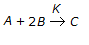a. 7/2 b. 7/4 c. 5/2 d. 5/4

 2. For a reaction of the following type, the rate of reaction (- rₓ) is given by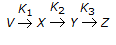a. (K₁+K₁)Cₓ b. (K₁+K₂+K₃)Cₓ c. K₁Cᵥ - K₂Cₓ d. (K₁-K₂)Cₓ
 3. In a consecutive reaction system given below, when E₁ is much greater than E₂, the yield of B increases with the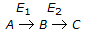a. increase of temperature. b. decrease of temperature. c. increase in initial concentration of A. d. decrease in initial concentration of A.
 4. For the irreversible elementary reactions in parallel like given below, the rate of disappearance of 'X' is equal to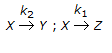a. Cᴀ(K₁+K₂) b. Cᴀ(K₁+K₂)/2 c. Cᴀ.K₁/2 d. Cᴀ.K₂/2
 5. For an isothermal variable volume batch reactor, the following relation is applicable for a first order irreversible reaction.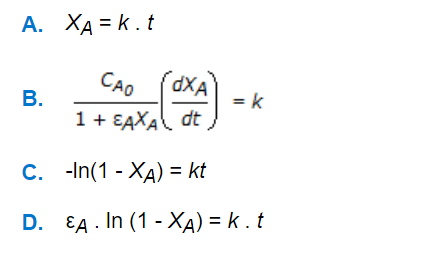a. A b. B c. C d. D